## Free Statistics

of Irreproducible Research!

Author's title
Author*The author of this computation has been verified*
R Software Modulerwasp_edauni.wasp
Title produced by softwareUnivariate Explorative Data Analysis
Date of computationWed, 24 Nov 2010 17:36:22 +0000
Cite this page as followsStatistical Computations at FreeStatistics.org, Office for Research Development and Education, URL https://freestatistics.org/blog/index.php?v=date/2010/Nov/24/t1290620079izpz7hdukekmm2z.htm/, Retrieved Sat, 23 Sep 2023 17:17:49 +0000
Statistical Computations at FreeStatistics.org, Office for Research Development and Education, URL https://freestatistics.org/blog/index.php?pk=100452, Retrieved Sat, 23 Sep 2023 17:17:49 +0000
QR Codes:Original text written by user:
IsPrivate?No (this computation is public)
User-defined keywords
Estimated Impact139
Family? (F = Feedback message, R = changed R code, M = changed R Module, P = changed Parameters, D = changed Data)
-     [Univariate Data Series] [Arabica Price in ...] [2008-01-05 23:43:18] [74be16979710d4c4e7c6647856088456]
- RMPD  [Univariate Explorative Data Analysis] [workshop 3 onderd...] [2010-11-24 13:40:45] [efd13e24149aec704f3383e33c1e842a]
-   PD      [Univariate Explorative Data Analysis] [autocorrelatie Di...] [2010-11-24 17:36:22] [c9d5faca36bd2ada281161976df30bf1] [Current]
-    D        [Univariate Explorative Data Analysis] [autocorrelatie wa...] [2010-11-24 17:39:02] [8ed0bd3560b9ca2814a2ed0a29182575]
Feedback Forum

Post a new message
Dataseries X:
142.500
148.185
138.712
164.365
168.000
181.425
197.324
235.942
265.748
290.284
305.831
300.444
313.157
339.266
348.618
392.328
404.302
422.683
358.412

 Summary of computational transaction Raw Input view raw input (R code) Raw Output view raw output of R engine Computing time 4 seconds R Server 'RServer@AstonUniversity' @ vre.aston.ac.uk

\begin{tabular}{lllllllll}
\hline
Summary of computational transaction \tabularnewline
Raw Input & view raw input (R code)  \tabularnewline
Raw Output & view raw output of R engine  \tabularnewline
Computing time & 4 seconds \tabularnewline
R Server & 'RServer@AstonUniversity' @ vre.aston.ac.uk \tabularnewline
\hline
\end{tabular}
%Source: https://freestatistics.org/blog/index.php?pk=100452&T=0

[TABLE]
[ROW][C]Summary of computational transaction[/C][/ROW]
[ROW][C]Raw Input[/C][C]view raw input (R code) [/C][/ROW]
[ROW][C]Raw Output[/C][C]view raw output of R engine [/C][/ROW]
[ROW][C]Computing time[/C][C]4 seconds[/C][/ROW]
[ROW][C]R Server[/C][C]'RServer@AstonUniversity' @ vre.aston.ac.uk[/C][/ROW]
[/TABLE]
Source: https://freestatistics.org/blog/index.php?pk=100452&T=0

Globally Unique Identifier (entire table): ba.freestatistics.org/blog/index.php?pk=100452&T=0

As an alternative you can also use a QR Code:

The GUIDs for individual cells are displayed in the table below:

 Summary of computational transaction Raw Input view raw input (R code) Raw Output view raw output of R engine Computing time 4 seconds R Server 'RServer@AstonUniversity' @ vre.aston.ac.uk

 Descriptive Statistics # observations 19 minimum 138.712 Q1 174.7125 median 290.284 mean 269.343473684211 Q3 343.942 maximum 422.683

\begin{tabular}{lllllllll}
\hline
Descriptive Statistics \tabularnewline
# observations & 19 \tabularnewline
minimum & 138.712 \tabularnewline
Q1 & 174.7125 \tabularnewline
median & 290.284 \tabularnewline
mean & 269.343473684211 \tabularnewline
Q3 & 343.942 \tabularnewline
maximum & 422.683 \tabularnewline
\hline
\end{tabular}
%Source: https://freestatistics.org/blog/index.php?pk=100452&T=1

[TABLE]
[ROW][C]Descriptive Statistics[/C][/ROW]
[ROW][C]# observations[/C][C]19[/C][/ROW]
[ROW][C]minimum[/C][C]138.712[/C][/ROW]
[ROW][C]Q1[/C][C]174.7125[/C][/ROW]
[ROW][C]median[/C][C]290.284[/C][/ROW]
[ROW][C]mean[/C][C]269.343473684211[/C][/ROW]
[ROW][C]Q3[/C][C]343.942[/C][/ROW]
[ROW][C]maximum[/C][C]422.683[/C][/ROW]
[/TABLE]
Source: https://freestatistics.org/blog/index.php?pk=100452&T=1

Globally Unique Identifier (entire table): ba.freestatistics.org/blog/index.php?pk=100452&T=1

As an alternative you can also use a QR Code:

The GUIDs for individual cells are displayed in the table below:

 Descriptive Statistics # observations 19 minimum 138.712 Q1 174.7125 median 290.284 mean 269.343473684211 Q3 343.942 maximum 422.683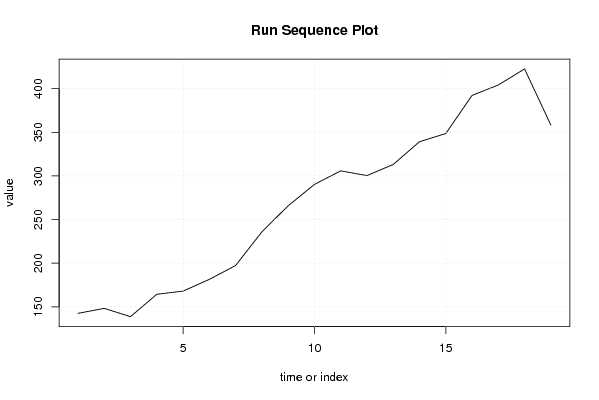PNG link Postscript link PDF link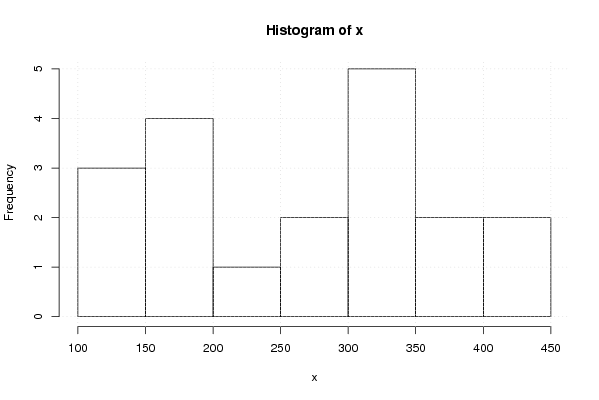PNG link Postscript link PDF link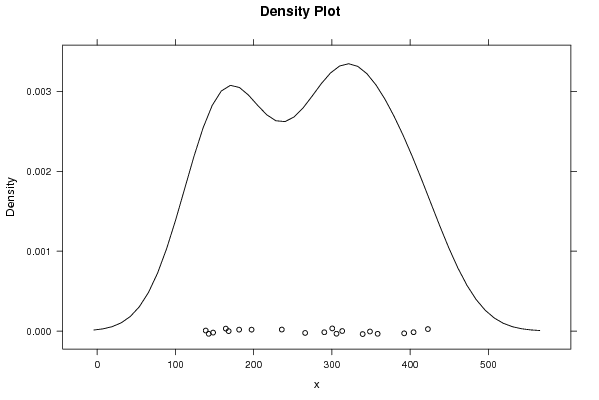PNG link Postscript link PDF link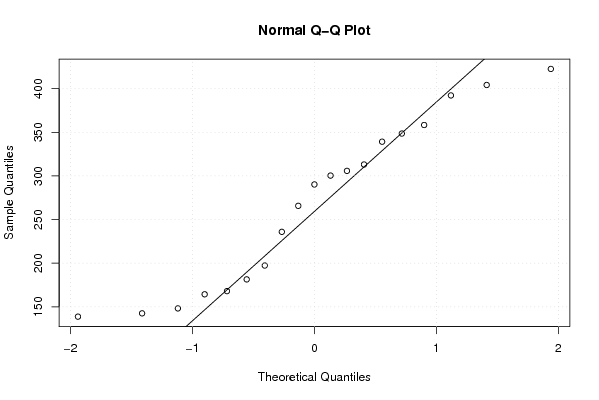PNG link Postscript link PDF link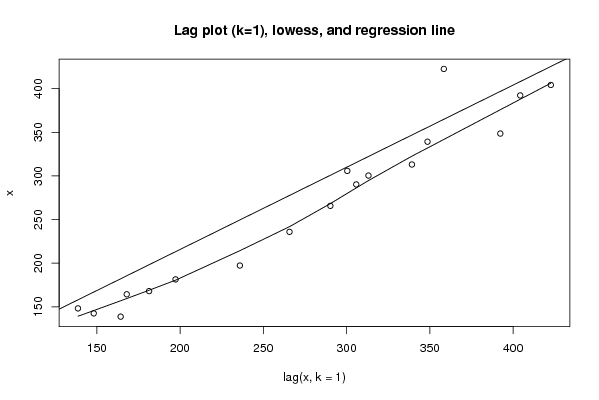PNG link Postscript link PDF link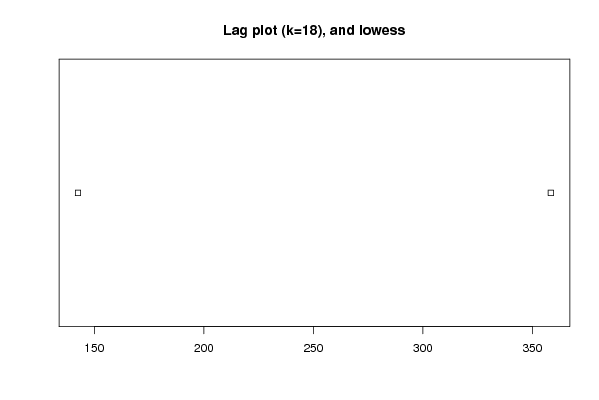PNG link Postscript link PDF link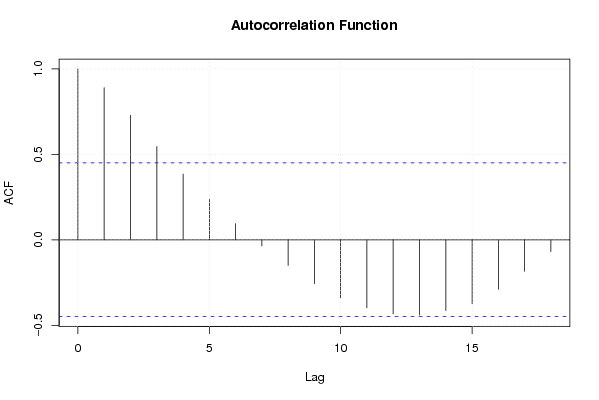PNG link Postscript link PDF link

Parameters (Session):
par1 = 0 ; par2 = 18 ;
Parameters (R input):
par1 = 0 ; par2 = 18 ;
R code (references can be found in the software module):
par1 <- as.numeric(par1)par2 <- as.numeric(par2)x <- as.ts(x)library(lattice)bitmap(file='pic1.png')plot(x,type='l',main='Run Sequence Plot',xlab='time or index',ylab='value')grid()dev.off()bitmap(file='pic2.png')hist(x)grid()dev.off()bitmap(file='pic3.png')if (par1 > 0){densityplot(~x,col='black',main=paste('Density Plot   bw = ',par1),bw=par1)} else {densityplot(~x,col='black',main='Density Plot')}dev.off()bitmap(file='pic4.png')qqnorm(x)qqline(x)grid()dev.off()if (par2 > 0){bitmap(file='lagplot1.png')dum <- cbind(lag(x,k=1),x)dumdum1 <- dum[2:length(x),]dum1z <- as.data.frame(dum1)zplot(z,main='Lag plot (k=1), lowess, and regression line')lines(lowess(z))abline(lm(z))dev.off()if (par2 > 1) {bitmap(file='lagplotpar2.png')dum <- cbind(lag(x,k=par2),x)dumdum1 <- dum[(par2+1):length(x),]dum1z <- as.data.frame(dum1)zmylagtitle <- 'Lag plot (k='mylagtitle <- paste(mylagtitle,par2,sep='')mylagtitle <- paste(mylagtitle,'), and lowess',sep='')plot(z,main=mylagtitle)lines(lowess(z))dev.off()}bitmap(file='pic5.png')acf(x,lag.max=par2,main='Autocorrelation Function')grid()dev.off()}summary(x)load(file='createtable')a<-table.start()a<-table.row.start(a)a<-table.element(a,'Descriptive Statistics',2,TRUE)a<-table.row.end(a)a<-table.row.start(a)a<-table.element(a,'# observations',header=TRUE)a<-table.element(a,length(x))a<-table.row.end(a)a<-table.row.start(a)a<-table.element(a,'minimum',header=TRUE)a<-table.element(a,min(x))a<-table.row.end(a)a<-table.row.start(a)a<-table.element(a,'Q1',header=TRUE)a<-table.element(a,quantile(x,0.25))a<-table.row.end(a)a<-table.row.start(a)a<-table.element(a,'median',header=TRUE)a<-table.element(a,median(x))a<-table.row.end(a)a<-table.row.start(a)a<-table.element(a,'mean',header=TRUE)a<-table.element(a,mean(x))a<-table.row.end(a)a<-table.row.start(a)a<-table.element(a,'Q3',header=TRUE)a<-table.element(a,quantile(x,0.75))a<-table.row.end(a)a<-table.row.start(a)a<-table.element(a,'maximum',header=TRUE)a<-table.element(a,max(x))a<-table.row.end(a)a<-table.end(a)table.save(a,file='mytable.tab')